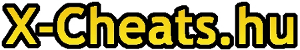Játék cheat kód, gépigény, és videó adatbázis

Pályakódok:
easy
L1 = utfooke
L2 = qbffekt
L3 = nnshufq
L4 = fjrlsrv
L5 = ggkbrab

medium
L1 = cxkbiff
L2 = krhfrch
L3 = kiiudkn
L4 = pqklcvy
L5 = eowlecf
L6 = blknlkp

hard
L1 = vicphnn
L2 = wpwqlan
L3 = posgsno
L4 = txqrowb
L5 = pexiwkq
L6 = pqqbgam
L7 = qxwwnte

very hard
L1 = uaaeksw
L2 = ivyfvnu
L3 = vyfukni
L4 = dunvexp
L5 = wlbsneo